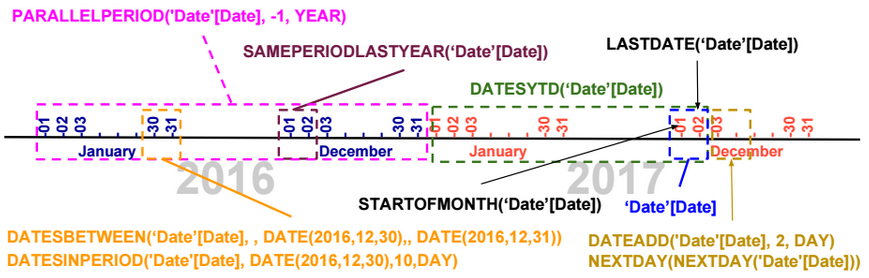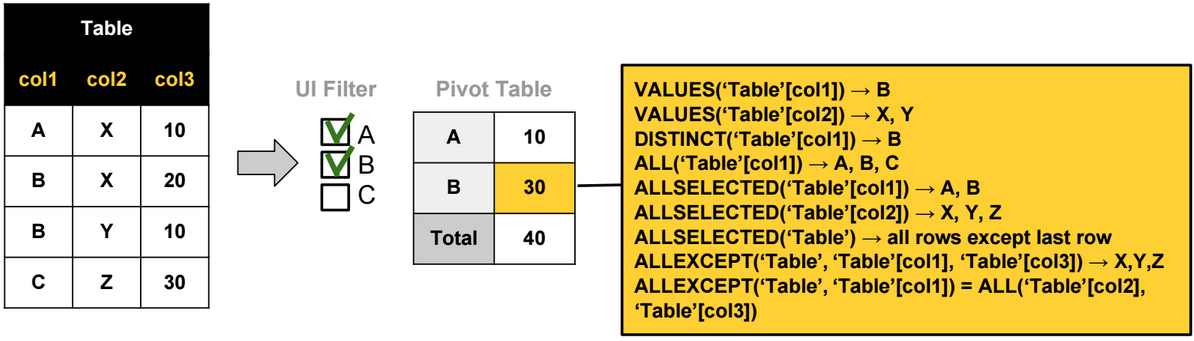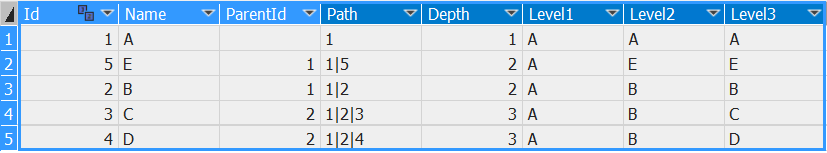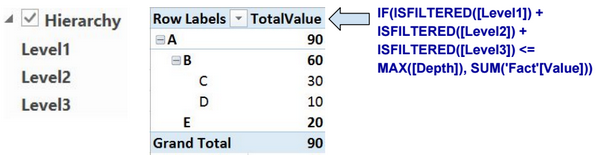# Time Intelligence Functions

The image below shows the output of each Time Intelligence Function when we pass as parameter a column �Date�[Date] containing two dates: 2017-12-01 and 2017-12-02.Using the CALCULATE function, we can evaluate a measure based on the result of a Time Intelligence function.

CALCULATE([TotalSales], DATESYTD(�Date�[Date]))

# Last Non Blank Function

LastNonBlank is a DAX function that returns the last value of a column for which an expression is not blank.

The DAX expression below returns the last date the measure Stock is not blank.

LASTNONBLANK(‘Date'[Date], [Stock])

# Calendar Functions

To generate a calendar, we can use the following functions: CALENDAR, CALENDARAUTO.

# Table FunctionsThe most used Table functions are:

ALL: Ignores any filters that might have been applied on a table or a column.

ALLSELECTED: Ignores any filters that might have been applied on a table or a column, but retains explicit filters (like Excel slicers). (In reality, this function removes all filter contexts generated by context transitions).

DISTINCT: Returns a one-column table that contains the distinct values from the specified column

VALUES: Returns a one-column table that contains the distinct values from the specified table or column.

ALLEXCEPT: Ignores any filters that might have been applied except on the specified columns. For example ALLEXCEPT(�Table�, Table�[col1]) is equivalent to ALL(�Table�[col2],�Table �[col3]).

ALLNOBLANKROW: Ignores any filters that might have been applied on a table or a column, returns all rows but the blank row, or all distinct values of a column but the blank row.

# Path Functions

Path functions are usually used when defining parent-child hierarchies. Below an example that shows how we can use these functions to create a parent-child hierarchy.

In the table below, we have a parent-child relationship based on two columns: Id and ParentId.The 5 calculated columns on the right are defined using the following DAX expressions:

 Calculated Column DAX Expression Path =PATH([Id],[ParentId]) Depth =PATHLENGTH([Path]) Level1 =IF([Depth] >=1, LOOKUPVALUE([Name],[Id], VALUE(PATHITEM([Path],1,1))), [Name]) Level2 =IF([Depth] >=2, LOOKUPVALUE([Name],[Id], VALUE(PATHITEM([Path],2,1))), [Name]) Level3 =IF([Depth] >=3, LOOKUPVALUE([Name],[Id], VALUE(PATHITEM([Path],3,1))), [Name])We use the columns Level1, Level2, and Level3 to define the parent-child hierarchy.

# LookUpValue Function

LookupValue function retrieves a lookup value from a lookup table.

# Count Functions

COUNT: Count numeric values.

COUNTA: Count rows different than blank.

COUNTBLANK: Count rows equal to blank.

COUNTROWS: COUNTA + COUNTBLANK.

DISTINCTCOUNT: Distinct count including Blank values.

# Other Functions

ISEMPTY

IFERROR, ISERROR

LEN, LEFT, RIGHT, SEARCH, SUBSTITUTE, REPLACE, FORMAT

DIVIDE

SWITCH

ISBLANK, ISTEXT, ISNONTEXT, ISNUMBER

CONCATENATEX

RANKX

ISFILTERED, ISCROSSFILTERED

HASONEVALUE

FLOOR, TRUNC, ROUNDDOWN, CEILING, MROUND, ROUNDUP

YEAR, MONTH, DAY

More details about these functions can be found here: https://msdn.microsoft.com/en-us/library/ee634396.aspx